# HBSE 8th Class Maths Solutions Chapter 13 Direct and Inverse Proportions Ex 13.1

Haryana State Board HBSE 8th Class Maths Solutions Chapter 13 Direct and Inverse Proportions Ex 13.1 Textbook Exercise Questions and Answers.

## Haryana Board 8th Class Maths Solutions Chapter 13 Direct and Inverse Proportions Exercise 13.1

Question 1.
Following are the car parking charges near a railway station upto
4 hours — Rs. 60
8 hours — Rs. 100
12 hours — Rs. 140
24 hours — Rs. 180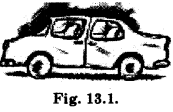Check if the parking charges are direct proportion to the parking time.
Solution:
Suppose the parking time of car is x hours and its charges in Rs. is y.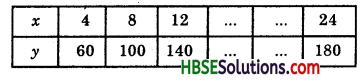If x and y are direct proportion, then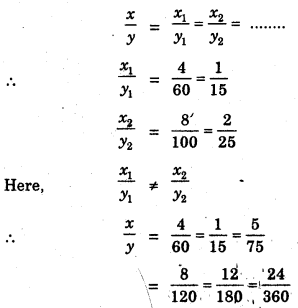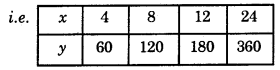Question 2.
A mixture of paint is prepared by mixing 1 part of red pigments with 8 parts of base. In the following table, find the parts of base that need to be added.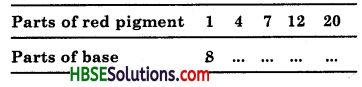Solution:
Let the parts of red pigment = x and
part of base = y.
∴ $$\frac{x}{y}$$ = $$\frac{1}{8}$$
$$\frac{x}{y}$$ = $$\frac{1}{32}$$ = $$\frac{4}{32}$$ = $$\frac{7}{56}$$ = $$\frac{12}{96}$$ = $$\frac{20}{160}$$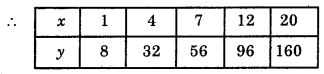Question 3.
In Question 2 above, if 1 part of a red pigment requires 75 mL of base, how much red pigment should we mix with 1800 mL of base ?
Solution:
Let red pigment = x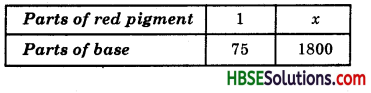∴ $$\frac{1}{75}$$ = $$\frac{x}{1800}$$
⇒ x × 75 = 1 × 1800
⇒ x = $$\frac{1800}{75}$$
∴ x = 24
Hence required red pigment = 24.

Question 4.
A machine in a soft drink factory fills 840 bottles in six hours. How many bottles will in fill in five hours ?
Solution:
Let the no. of bottles = x
Number of time and number of bottles vary directly.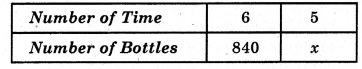Now, $$\frac{6}{840}$$ = $$\frac{5}{x}$$
⇒ 6 × x = 5 × 840
⇒ x = $$\frac{5×840}{6}$$
⇒ x = 700
Hence, required bottles = 700.Question 5.
A photograph of a bacteria enlarged 50,000 times attains a length of 5 cm as shown in the diagram. What is the actual length of the bacteria ? If the photograph is enlarged 20,000 times only, what would be enlarged length ?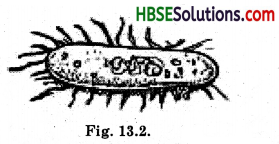Solution:
Let the length of bacteria = x. Length of bacteria and enlarged times of bacteria vary directly.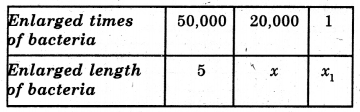Now, $$\frac{50,000}{5}$$ = $$\frac{20,000}{x}$$
⇒ 50,000 × x = 5 × 20,000
⇒ x = $$\frac{5×20,000}{50,000}$$
⇒ x = 2
∴ Enlarged length = 2 cm
and Actual length, x1 = $$\frac{2}{20,000}$$
= $$\frac{1}{10,000}$$ = 0.0001 cm.

Question 6.
In a model of a ship, the mast is 9 cm high, while the mast of the actual ship is 12 m high. If the length of the ship is 28 m, how long is the model ship ?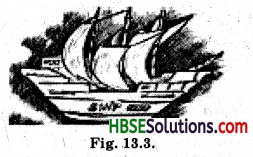Solution:
Try Yourself.Question 7.
Suppose 2 kg of sugar contains 9 × 106 crystals. How many sugar crystals are there in
(i) 5 kg of sugar ?
(ii) 1.2 kg of sugar ?
Solution:
Let 5 kg of sugar contains = x crystals
and 1.2 kg of sugar contains = y crystals.
Sugar and crystals vary directly.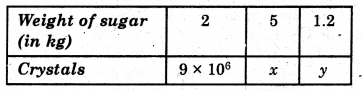Now, (i) $$\frac{2}{9 \times 10^{6}}$$ = $$\frac{5}{x}$$
⇒ 2 × x = 5 × 9 × 10
⇒ x = $$\frac{5 \times 9 \times 10 \times 10^{5}}{2}$$
⇒ x = 225 × 105
= 2.25 × 107
∴ 5 kg of sugar contains 2.25 × 107 crystals.

(ii) $$\frac{2}{9 \times 10^{6}}$$ = $$\frac{1.2}{y}$$
⇒ 2 × y = 1.2 × 9 × 106
⇒ y = $$\frac{1.2 \times 9 \times 10 \times 10^{5}}{2}$$
= 54 × 106
= 5.4 × 106
∴ 1.2 kg of sugar contains 5.4 × 106 crystals.

Question 8.
Rashmi has a road map with a scale of 1 cm representing 18 km. She drives on a road for 72 km. What would be her distance covered in the map ?
Solution:
Let the distance covered in the map = x.
Map with a scale and distance covered in the map vary directly.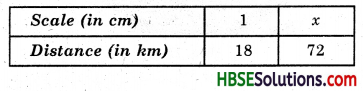Now, $$\frac{1}{18}$$ = $$\frac{x}{72}$$
⇒ 18 × x = 72 × 1
⇒ x = $$\frac{72}{18}$$ = 4
Hence required distance covered in the map = 4 cm.Question 9.
A 5 m 60 cm high vertical pole casts a shadow 3 m 20 cm long. Find at the same time
(i) the length of the shadow cast by another pole 10 m 50 cm high,
(ii) the height of a pole which casts a shadow 5 m long.
Solution:
Let the length of shadow = x
and the height of a pole = y
Length of the shadow and height of pole vary directly.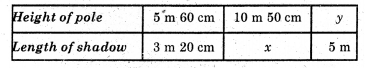Now, (i) $$\frac{5.60}{3.20}$$ = $$\frac{10.50}{x}$$
⇒ 5.60 × x = 3.20 × 10.50
⇒ x = $$\frac{3.20 \times 10.50}{5.60}$$ = 6 m
Hence required length of shadow 6m.

(ii) $$\frac{5.60}{3.20}$$ = $$\frac{y}{5}$$
⇒ 3.20 × y = 5.60 × 5
⇒ y = $$\frac{5.60 \times 5}{3.20}$$ = 8.75
Hence required height of pole = 8.75 m or 8 m 75 cm.

Question 10.
A loaded truck travels 14 km in 25 minutes. If the speed remains the same, how far can it travel in 5 hours ?
Solution:
60 minutes = 1 hour
∴ 25 minutes = $$\frac{1}{60}$$ × 25 = $$\frac{5}{12}$$ hour
Let distance of travelling truck = x
Distance of travelling truck and time vary directly.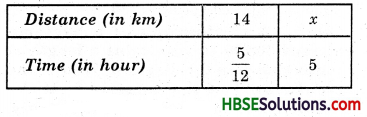Now, $$\frac{14}{5 / 12}$$ = $$\frac{x}{5}$$
⇒ $$\frac{5}{12}$$ × x = 14 × 5
⇒ x = $$\frac{14 \times 5}{5 / 12}$$ = $$\frac{14 \times 5 \times 12}{5}$$
⇒ x = 168 km
Hence distance of travelling truck = 168 km.